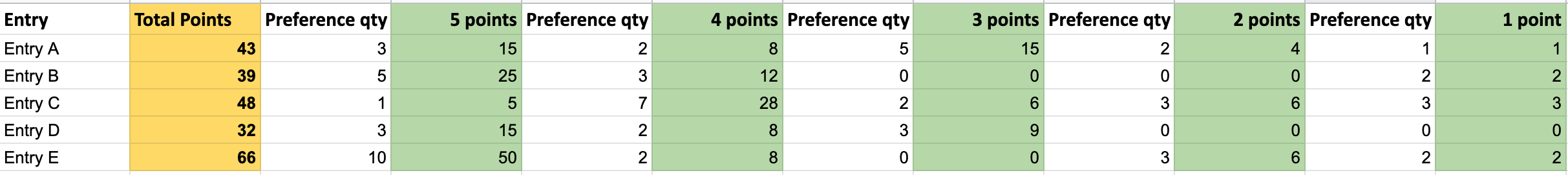# How to calculate a ranked list when using Top pick

The Top pick judging mode in Award Force does not produce a ranked list of winners but a group of winners in no particular order. To generate a ranked list, the Borda count method needs to be used outside of Award Force with your exported results in Excel.

## Borda count

Here is an explanation of the standard Borda count method, where points are allocated to entries based on the number of 1st preferences, 2nd preferences, 3rd preferences (and so on).

Essentially, each preference is given a score value, for this example, we'll work with finding the top 5 entries based on user preferences. Based on the number of ranked winners we want, we start by assigning the lowest preference (i.e. 5th) and lowest point value (i.e. 1). At the other end of the scale, we give the 1st preference the highest point value (i.e. 5). So, points per preference would be:

• 1st preference = 5 points
• 2nd preference = 4 points
• 3rd preference = 3 points
• 4th preference = 2 points
• 5th preference = 1 point

So, if an entry had the following distribution of preferences:

1st preference
2nd preference
3rd preference
4th preference
5th
preference
Entry A  3  2  5  2  1

Then the points would be:

1st preference
2nd preference
3rd preference
4th preference
5th
preference
Entry A  3 x 5 points  2 x 4 points  5 x 3 points  2 x 2 points  1 x 1 point
=15  =8  =15  =4  =1

Therefore, Entry A would have scored a total of: 15 + 8 + 15 + 4 + 1 = 43 points.

Let's look at this with a larger dataset:

1st preference

(5 points)

2nd preference

(4 points)

3rd preference

(3 points)

4th preference

(2 points)

5th preference

(1 point)

Total points

Entry A  3  2  5  2  1  =43
3x5 = 15  2x4 = 8  5x3 = 15  2x2 = 4  1x1 = 1
Entry B  5  3  0  0  2  =39
5x5 = 25  3x4 = 12  0x3 = 0  0x2 = 0  2x1 = 2
Entry C  1   7  2  3  3  =48
1x5 = 5  7x4 = 28  2x3 = 6  3x2 = 6  3x1 = 3
Entry D  3  2  3  0  0  =32
3x5 = 15  2x4 = 8  3x3 = 6  0x2 = 2  0x1 = 0
Entry E  10  2  0  3  2 =66
10x5 = 50  2x4 = 8  0x3 = 0  3x2 = 6  2x1 = 2

Based on the total points for each entry, this would be the ranked list of entries from 1st to 5th:

• 1st: Entry E, 66 points
• 2nd: Entry C, 48 points
• 3rd: Entry A, 43 points
• 4th: Entry B, 39 points
• 5th: Entry D, 32 points

## How to put Borda count into practice

1. In the Manage workspace, go to Judging > Leaderboard
2. Open the Export drop-down